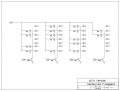# Updated Game Circuit In Progress

#### djsfantasi

Joined Apr 11, 2010
7,919
I'm updating an old project of mine (link to Game Show circuit). I want to add some wireless keychain remotes to trigger the lockout. And I want to have a better output than a single LED. I decided to use large (1"H) 7-segment displays, hardwiring each of the four to display a number from 1 to 4.

I was hoping that I could get a second pair of eyes, to review my calculations. I haven't picked out the final display yet, but used the following values to make some initial calculations:
Iseg 0.020 A
Vcc 9 VDC
Vf 1.8 VDC​

Here is a schematic of the design for the displays.Based on the above assumptions, I determined that I needed a 390 resistor for each segment.

The numbers 1 - 4 have varying number of segments lit. from 2 segments for a '1', '4' requires 4 segments and '2' and '3' require 5 segments lit. From this, I calculated the total current for each digit. Which led me to determine the value of the base resistors (at 1/10th the total current).

Below is a screenshot of the Excel spreadsheet I used.To calculate the LED resistor, I used $$R_{LED}=\frac{V_{cc}-V_{f}}{I_{seg}}$$
To calculate the base resistor, I used $$V_{base}=V_{cc}-0.6$$
Then, I calculate the base resistor as follows: $$R_{base}=\frac{V_{base}}{I_{base}}$$,
where $$I_{base}=I_{total}/10$$
$$I_{total}=n*I_{segment}$$

Can anyone comment on my approach and confirm my calculations and design?

Last edited:

#### wayneh

Joined Sep 9, 2010
17,155
Looks fine to me.

A Vf of 1.8V is low, so these must be red LEDs? You might consider whether they really need 20mA. You'll easily see them at just 5mA, and they'll last longer. Play around and see, if you haven't already.

I assume you don't plan to use a 9V battery for this. I was going to point out how much they will sag in voltage with age, but you must be planning another power source.

You should also consider putting LEDs in series. If you really have a full 9V and only need a Vf of 1.8V, you should be able to put 4 in series, maybe even 5. You wouldn't need as many resistors, the current through the LEDs in any one string will be identical, and there will be less load on the power supply.

•djsfantasi

#### MMcLaren

Joined Feb 14, 2010
853
Just curious... have you considered using a single display instead of four separate displays?

Mike

•djsfantasi

#### djsfantasi

Joined Apr 11, 2010
7,919
I considered putting the segments in series, but they're packaged common cathode. I don't know if I can find a 1"+ tall display with separate pins per segment.

I did consider a single display, but liked the look of multiple displays, where each team is identified by its number and position. The game logic outputs one pin per team. Currently, it drives an LED. I am retrofitting to this logic. I could create a diode matrix as an decoder... But I'd lose the positional aspect.

The existing circuit runs on a 9 VDC battery. I plan on driving the circuit and LEDs with 12 VDC. The CD4000 logic circuits are good to 15 VDC. I have a wall wart from a piece of computer equipment rated at 12 VDC. Change one value in the spreadsheet will calculate the new resistor values. (I found an Excel add-in that provides a function to convert a calculated value to standard resistor values)

I calculated with the LED current at 20 ma, because I want the brightness; the displays should be readable across a classroom. They are rated to 30 ma maximum. With a standard E12 resistor,
Calculated for 20 ma, they run at or about 18 ma.

#### wayneh

Joined Sep 9, 2010
17,155
If space is an issue, you might consider a MOSFET array for your transistors. You wouldn't need all the base resistors and you'd have just the one IC. Slightly more efficient also because of the removal of base current.